# Electric Charge and Electric Field AP Physics Chapter

• Slides: 27Electric Charge and Electric Field AP Physics Chapter 16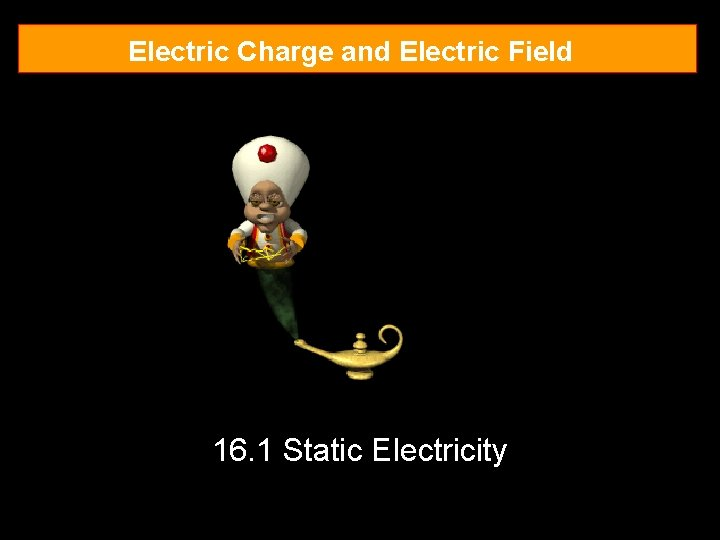Electric Charge and Electric Field 16. 1 Static Electricity16. 1 Static Electricity Static electricity – not moving Two types of charge positive (+) when electrons are lost negative (-) when electrons are gained Objects can gain charges by rubbing 16. 1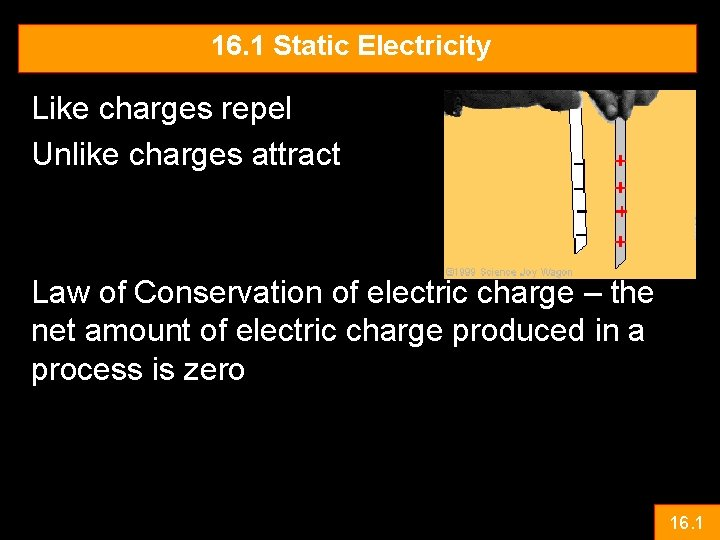16. 1 Static Electricity Like charges repel Unlike charges attract Law of Conservation of electric charge – the net amount of electric charge produced in a process is zero 16. 1Electric Charge and Electric Field 16. 2 Electric Charge in the Atom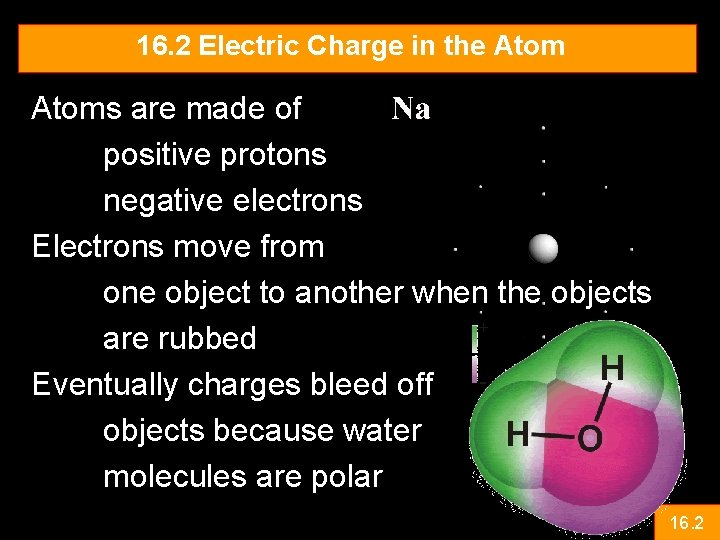16. 2 Electric Charge in the Atoms are made of positive protons negative electrons Electrons move from one object to another when the objects are rubbed Eventually charges bleed off objects because water molecules are polar 16. 2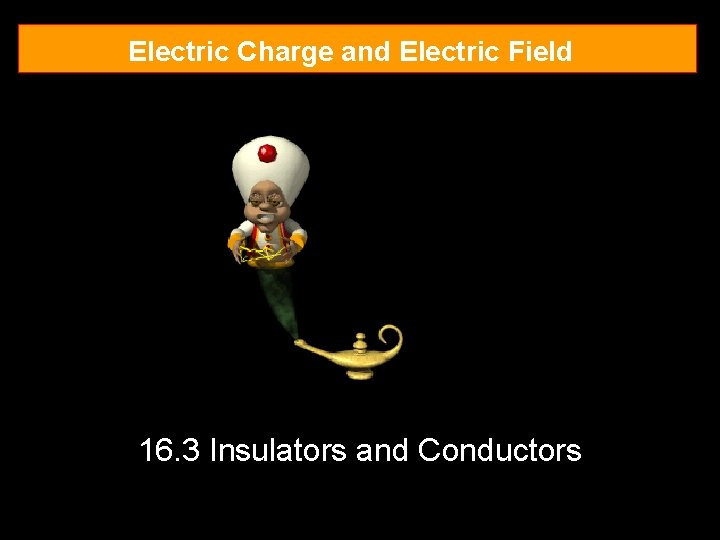Electric Charge and Electric Field 16. 3 Insulators and Conductors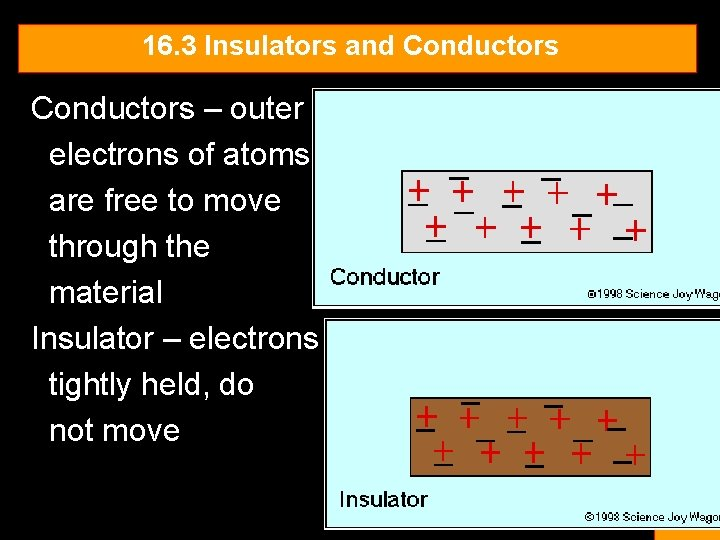16. 3 Insulators and Conductors – outer electrons of atoms are free to move through the material Insulator – electrons tightly held, do not move 16. 316. 3 Insulators and Conductors Semiconductors – conduct electricity under some circumstances, don’t under other conditions 16. 3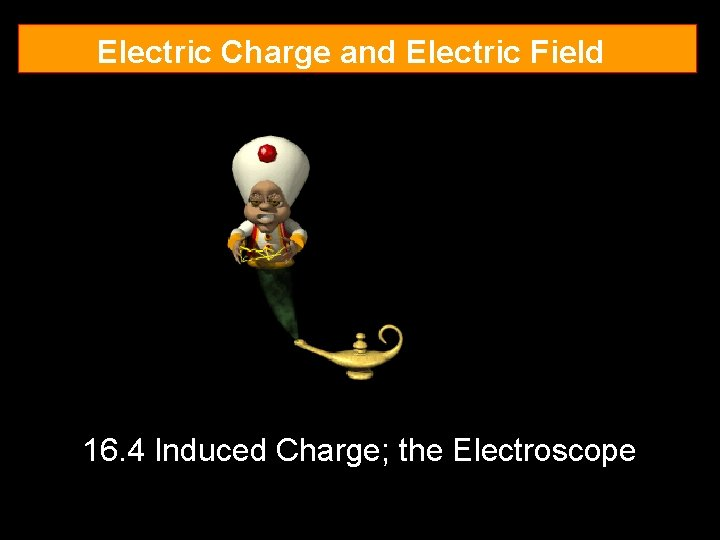Electric Charge and Electric Field 16. 4 Induced Charge; the Electroscope16. 4 Induced Charge; the Electroscope Induction – charging without contact Object is brought near a charged object Electrons move Object is grounded An electroscope measures if an object has a charge on it 16. 4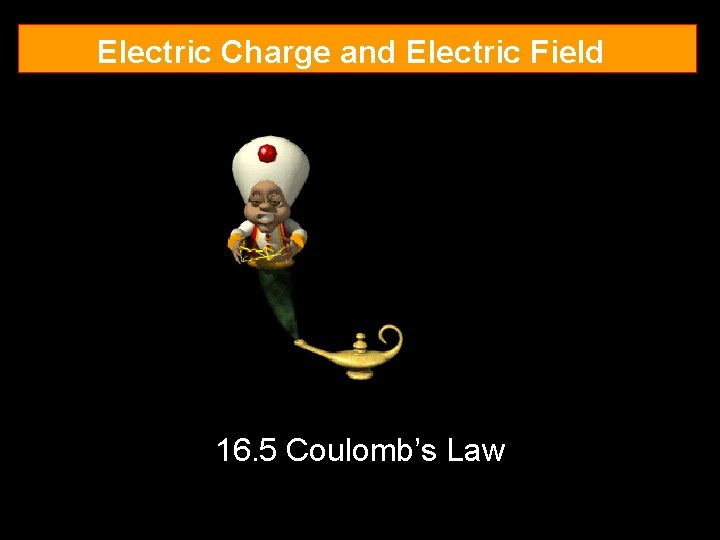Electric Charge and Electric Field 16. 5 Coulomb’s Law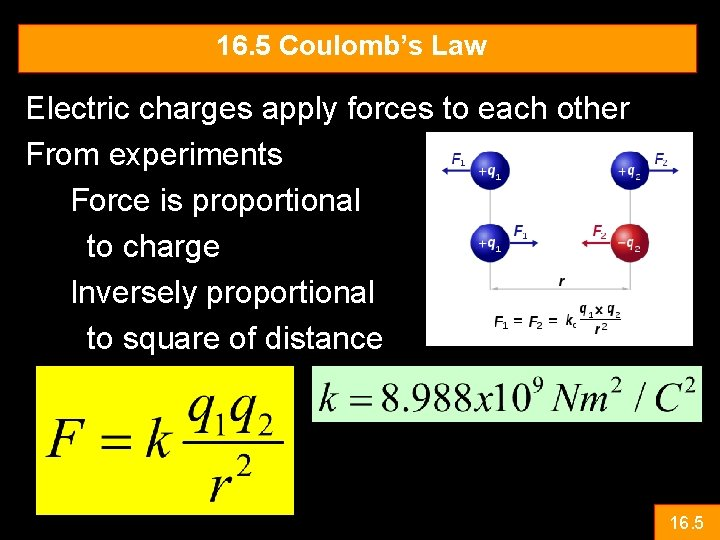16. 5 Coulomb’s Law Electric charges apply forces to each other From experiments Force is proportional to charge Inversely proportional to square of distance 16. 5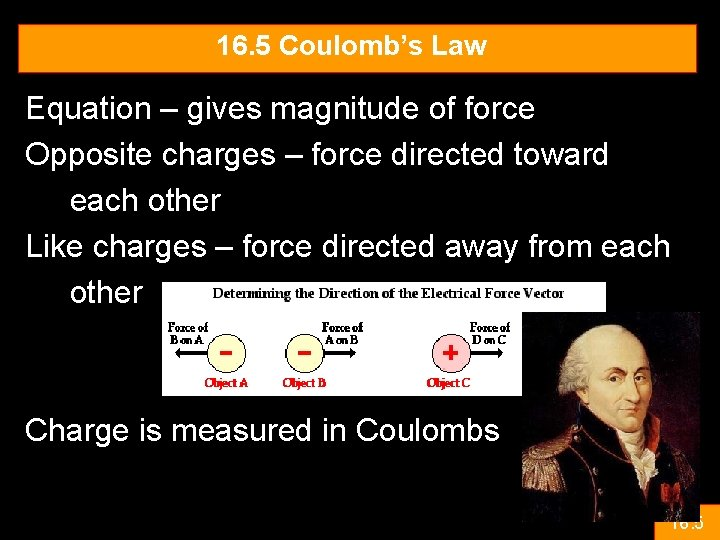16. 5 Coulomb’s Law Equation – gives magnitude of force Opposite charges – force directed toward each other Like charges – force directed away from each other Charge is measured in Coulombs 16. 516. 5 Coulomb’s Law 1 Coulomb is the amount of charge, that if placed 1 m apart would result in a force of 9 x 109 N Charges are quantized – that is they come in discrete values The constant k relates to the constant called the permittivity of free space 16. 5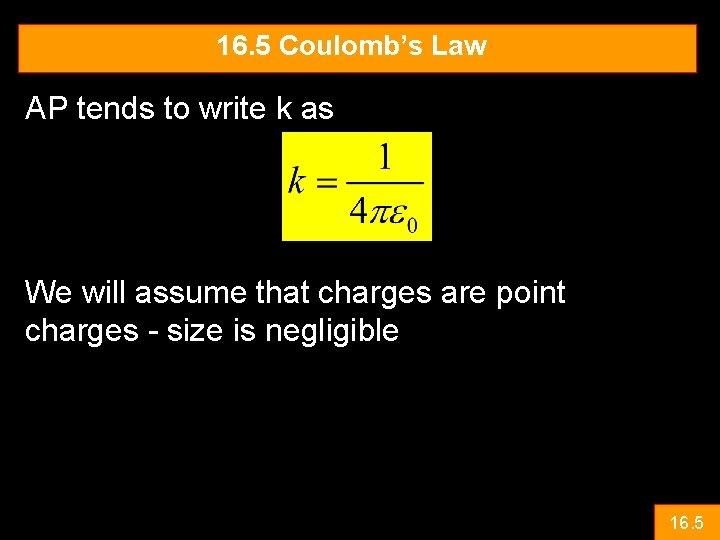16. 5 Coulomb’s Law AP tends to write k as We will assume that charges are point charges - size is negligible 16. 5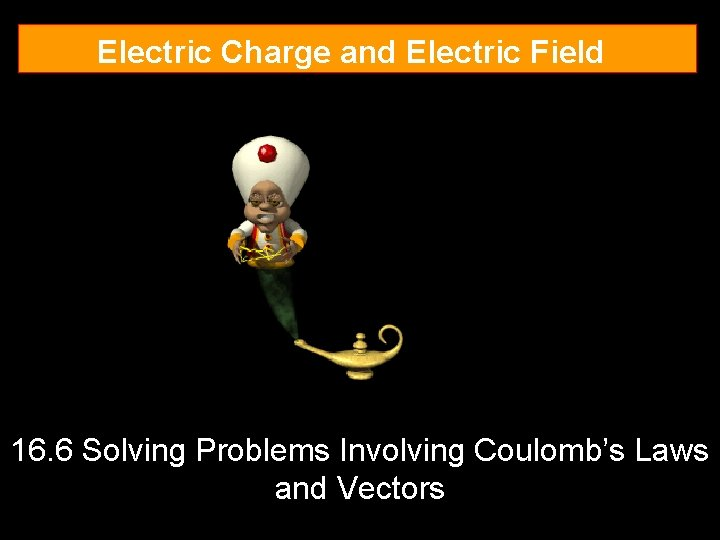Electric Charge and Electric Field 16. 6 Solving Problems Involving Coulomb’s Laws and Vectors16. 6 Coulomb’s Law and Vectors Principle of Superposition – Electrostatic forces are added just like any other force As vectors 16. 6Electric Charge and Electric Field 16. 7 The Electric Field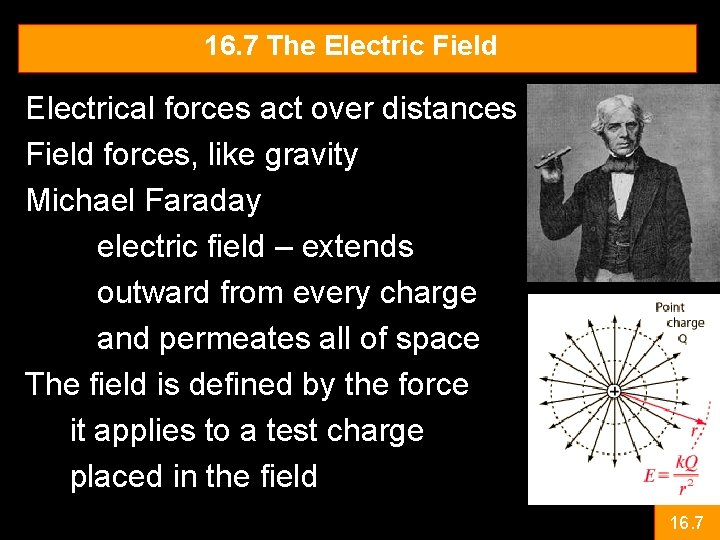16. 7 The Electric Field Electrical forces act over distances Field forces, like gravity Michael Faraday electric field – extends outward from every charge and permeates all of space The field is defined by the force it applies to a test charge placed in the field 16. 716. 7 The Electric Field The Electric field would then be Or q is the test charge We can also say that Remember that E is independent of the test charge. The electric field is also a vector (free body diagrams are probably a good idea) 16. 7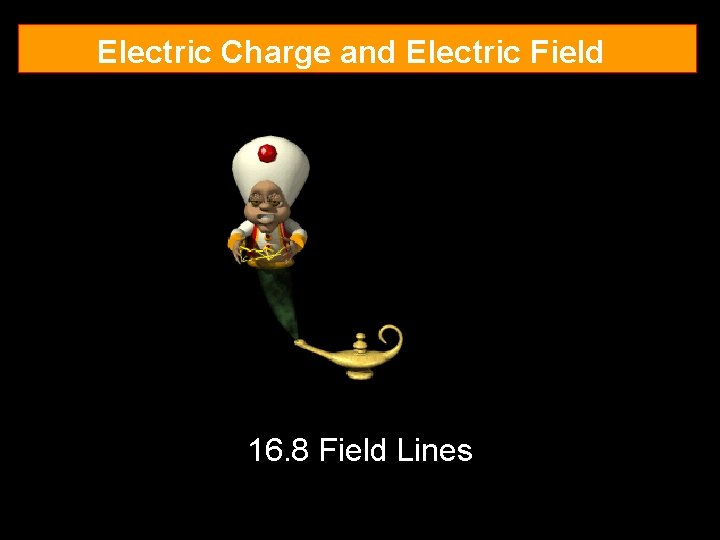Electric Charge and Electric Field 16. 8 Field Lines16. 8 Field Lines To visualize electric fields Draw electric field lines Direction of the lines is the direction of force on a positive test charge The density of the lines indicates relative strength of the field Note: the field density increase as you get closer 16. 8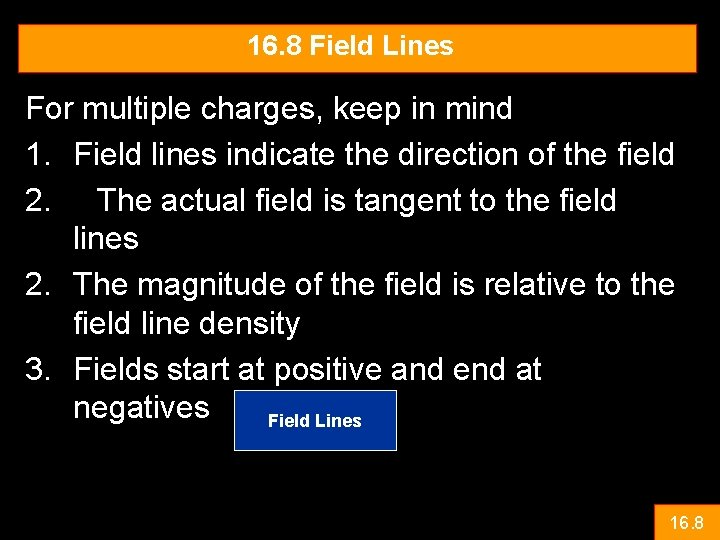16. 8 Field Lines For multiple charges, keep in mind 1. Field lines indicate the direction of the field 2. The actual field is tangent to the field lines 2. The magnitude of the field is relative to the field line density 3. Fields start at positive and end at negatives Field Lines 16. 8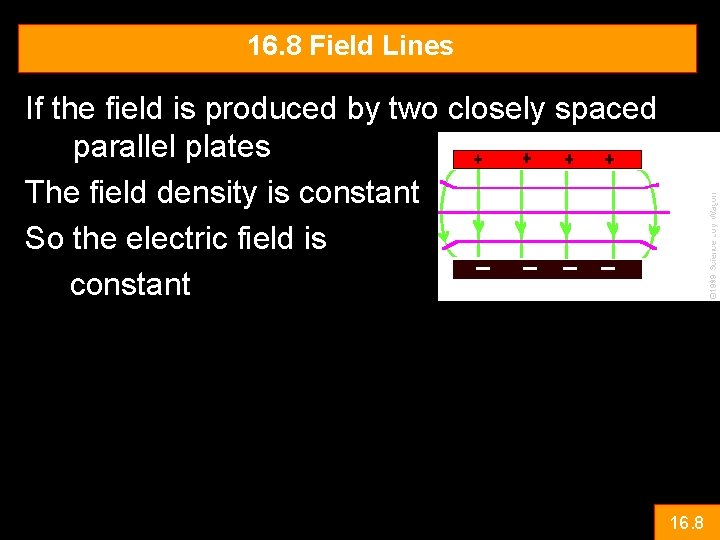16. 8 Field Lines If the field is produced by two closely spaced parallel plates The field density is constant So the electric field is constant 16. 8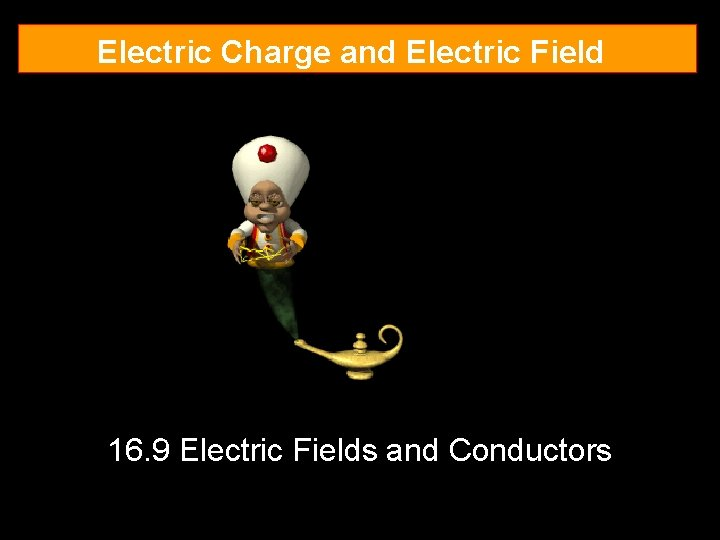Electric Charge and Electric Field 16. 9 Electric Fields and Conductors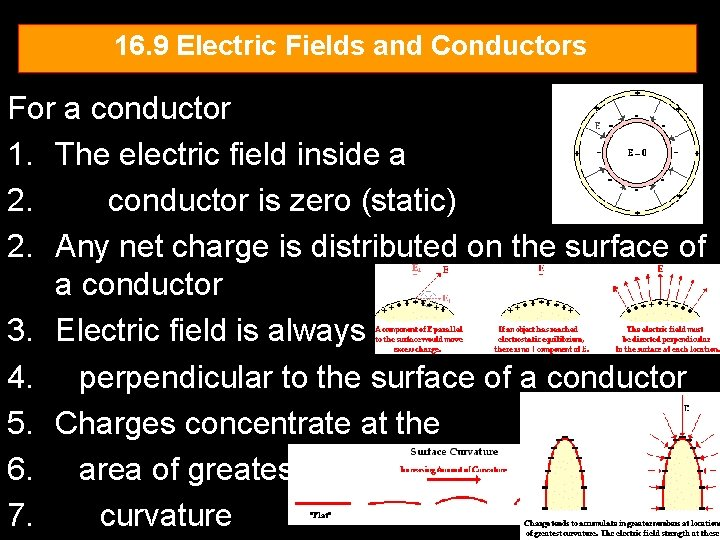16. 9 Electric Fields and Conductors For a conductor 1. The electric field inside a 2. conductor is zero (static) 2. Any net charge is distributed on the surface of a conductor 3. Electric field is always 4. perpendicular to the surface of a conductor 5. Charges concentrate at the 6. area of greatest 7. curvature 16. 9